Updating search results...

# 16 Results

View
Selected filters:
• Square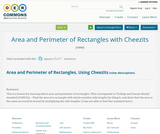Unrestricted Use
CC BY
Rating
4.0 stars

This is a lesson for learning about area and perimeter of rectangles.

This corresponds to "College and Career Ready" standard (3.MD.7a) -- Find the area of a rectangle with whole-number side lengths by tiling it, and show that the area is the same as would be found by multiplying the side lengths.
(I was not able to find that standard here.)

Subject:
Mathematics
Material Type:
Lesson Plan
Author:
Susan Jones
01/28/2017
Remix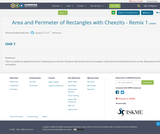Unrestricted Use
CC BY
Rating
0.0 stars

This is a hands-on guided lesson for relating area to factors. Students will model area of rectangles using Cheez-Its and define factors as the dimensions of the rectangles.

Subject:
Mathematics
Material Type:
Lesson Plan
Author:
Donna Parrish
08/08/2019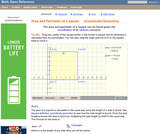Rating
0.0 stars

An interactive applet and associated web page showing how to find the area and perimeter of a square from the coordinates of its vertices. The square can be either parallel to the axes or rotated. The grid and coordinates can be turned on and off. The area and perimeter calculation can be turned off to permit class exercises and then turned back on the verify the answers. The applet can be printed as it appears on the screen to make handouts. The web page has a full description of the method for determining area and perimeter, a worked example and has links to other pages relating to coordinate geometry. Applet can be enlarged to full screen size for use with a classroom projector. This resource is a component of the Math Open Reference Interactive Geometry textbook project at http://www.mathopenref.com.

Subject:
Geometry
Material Type:
Simulation
Provider:
Math Open Reference
Author:
John Page
02/16/2011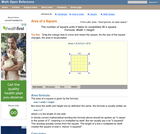Rating
0.0 stars

An interactive applet and associated web page that demonstrate the area of a square. The applet shows a square with all vertices draggable. As you drag any one, the area id continuously calculated and shown on the applet. The square is filled with a unit grid to allow class estimation of area. The displayed calculation can be turned off. Applet can be enlarged to full screen size for use with a classroom projector. This resource is a component of the Math Open Reference Interactive Geometry textbook project at http://www.mathopenref.com.

Subject:
Geometry
Material Type:
Simulation
Provider:
Math Open Reference
Author:
John Page
02/16/2011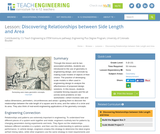Educational Use
Rating
0.0 stars

Through this lesson and its two associated activities, students are introduced to the use of geometry in engineering design, and conclude by making scale models of objects of their choice. The practice of developing scale models is often used in engineering design to analyze the effectiveness of proposed design solutions. In this lesson, students complete fencing (square) and fire pit (circle) word problems on two worksheets—which involves side and radius dimensions, perimeters, circumferences and areas—guiding them to discover the relationships between the side length of a square and its area, and the radius of a circle and its area. They also think of real-world engineering applications of the geometry concepts.

Subject:
Mathematics
Geometry
Measurement and Data
Material Type:
Lesson
Provider:
TeachEngineering
Author:
Aaron Lamplugh
Malinda Zarske
Nathan Coyle
Russell Anderson
Ryan Sullivan
02/07/2017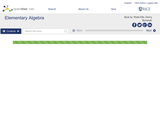Unrestricted Use
CC BY
Rating
0.0 stars

Elementary Algebra is a work text that covers the traditional topics studied in a modern elementary algebra course. It is intended for students who (1) have no exposure to elementary algebra, (2) have previously had an unpleasant experience with elementary algebra, or (3) need to review algebraic concepts and techniques.

Subject:
Algebra
Material Type:
Full Course
Textbook
Provider:
Rice University
Provider Set:
OpenStax CNX
Author:
Denny Burzynski
08/16/2010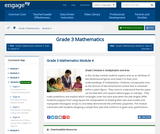Conditional Remix & Share Permitted
CC BY-NC-SA
Rating
0.0 stars

In this 20-day module students explore area as an attribute of two-dimensional figures and relate it to their prior understandings of multiplication. Students conceptualize area as the amount of two-dimensional surface that is contained within a plane figure.  They come to understand that the space can be tiled with unit squares without gaps or overlaps.  They make predictions and explore which rectangles cover the most area when the side lengths differ.  Students progress from using square tile manipulatives to drawing their own area models and manipulate rectangular arrays to concretely demonstrate the arithmetic properties. The module culminates with students designing a simple floor plan that conforms to given area specifications.

Subject:
Measurement and Data
Material Type:
Module
Provider:
New York State Education Department
Provider Set:
EngageNY
10/18/2013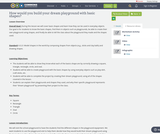Only Sharing Permitted
CC BY-NC-ND
Rating
0.0 stars

Subject:
Geometry
Material Type:
Lesson Plan
Author:
summer dickerhoof
12/07/2017
Remix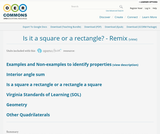Conditional Remix & Share Permitted
CC BY-NC
Rating
0.0 stars

Students will look at examples and non-examples of squares and rectangles to determine properties of the two shapes. Students practice drawing the shapes with specific dimensions and building up to is a square also a rectangle and is a rectangle also a square?

Subject:
Mathematics
Material Type:
Activity/Lab
Lesson Plan
Author:
Tres Wells
08/08/2019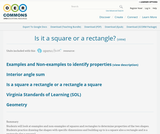Conditional Remix & Share Permitted
CC BY-NC
Rating
0.0 stars

Students will look at examples and non-examples of squares and rectangles to determine properties of the two shapes. Students practice drawing the shapes with specific dimensions and building up to is a square also a rectangle and is a rectangle also a square?

Subject:
Mathematics
Material Type:
Activity/Lab
Lesson Plan
Author:
Peter Fiddner
02/13/2016Conditional Remix & Share Permitted
CC BY-NC
Rating
0.0 stars

Subject:
Mathematics
Material Type:
Full Course
Provider:
Pearson
10/06/2016Rating
0.0 stars

Surface Area and Volume

Type of Unit: Conceptual

Prior Knowledge

Students should be able to:

Identify rectangles, parallelograms, trapezoids, and triangles and their bases and heights.
Identify cubes, rectangular prisms, and pyramids and their faces, edges, and vertices.
Understand that area of a 2-D figure is a measure of the figure's surface and that it is measured in square units.
Understand volume of a 3-D figure is a measure of the space the figure occupies and is measured in cubic units.

Lesson Flow

The unit begins with an exploratory lesson about the volumes of containers. Then in Lessons 2–5, students investigate areas of 2-D figures. To find the area of a parallelogram, students consider how it can be rearranged to form a rectangle. To find the area of a trapezoid, students think about how two copies of the trapezoid can be put together to form a parallelogram. To find the area of a triangle, students consider how two copies of the triangle can be put together to form a parallelogram. By sketching and analyzing several parallelograms, trapezoids, and triangles, students develop area formulas for these figures. Students then find areas of composite figures by decomposing them into familiar figures. In the last lesson on area, students estimate the area of an irregular figure by overlaying it with a grid. In Lesson 6, the focus shifts to 3-D figures. Students build rectangular prisms from unit cubes and develop a formula for finding the volume of any rectangular prism. In Lesson 7, students analyze and create nets for prisms. In Lesson 8, students compare a cube to a square pyramid with the same base and height as the cube. They consider the number of faces, edges, and vertices, as well as the surface area and volume. In Lesson 9, students use their knowledge of volume, area, and linear measurements to solve a packing problem.

Subject:
Mathematics
Geometry
Material Type:
Unit of Study
Provider:
Pearson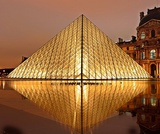Conditional Remix & Share Permitted
CC BY-NC
Rating
0.0 stars

Lesson OverviewStudents use scissors to transform a net for a unit cube into a net for a square pyramid. They then investigate how changing a figure from a cube to a square pyramid affects the number of faces, edges, and vertices and how it changes the surface area and volume.Key ConceptsA square pyramid is a 3-D figure with a square base and four triangular faces.In this lesson, the net for a cube is transformed into a net for a square pyramid. This requires cutting off one square completely and changing four others into isosceles triangles.It is easy to see that the surface area of the pyramid is less than the surface area of the cube, because part of the cube's surface is cut off to create the pyramid. Specifically, the surface area of the pyramid is 3 square units, and the surface area of the cube is 6 square units. Students will be able to see visually that the volume of the pyramid is less than that of the cube.Students consider the number of faces, vertices, and edges of the two figures. A face is a flat side of a figure. An edge is a segment where 2 faces meet. A vertex is the point where three or more faces meet. A cube has 6 faces, 8 vertices, and 12 edges. A square pyramid has 5 faces, 5 vertices, and 8 edges.Goals and Learning ObjectivesChange the net of a cube into the net of a pyramid.Find the surface area of the pyramid.

Subject:
Geometry
Material Type:
Lesson Plan
Provider:
Pearson
09/21/2015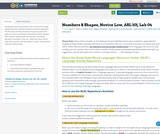Conditional Remix & Share Permitted
CC BY-NC-SA
Rating
0.0 stars

During the warm-up students will review how to sign shapes and the cardinal numbers from the slideshow. For the main activity, students will pair up and each grab a picture card without showing it to their partner. One student will describe the picture card being specific to location, color, etc, while the other draws what their partner just described to them. The partners will then switch roles.

Subject:
Languages
Material Type:
Activity/Lab
Author:
Amber Hoye
Megan McAllister
Delaney Lyon
Sarra Foerster
Izabelle Finner
Camille Daw
Mimi Fahnstrom
04/07/2020
Remix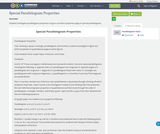Unrestricted Use
CC BY
Rating
0.0 stars

Students investigate parallelogram properties to figure out which properties apply to special parallelograms.

Subject:
Mathematics
Material Type:
Activity/Lab
Author:
Becky Lancaster
03/23/2020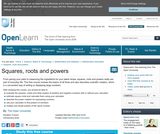Conditional Remix & Share Permitted
CC BY-NC-SA
Rating
0.0 stars

From paving your patio to measuring the ingredients for your latest recipe, squares, roots and powers really are part of everyday life. This unit reviews the basics of all three and also describes scientific notation, which is a convenient way of writing or displaying large numbers.

Subject:
Mathematics
Material Type:
Activity/Lab